# Division Table PrintableOtherMar 18, 2021By PrintableeNo Comment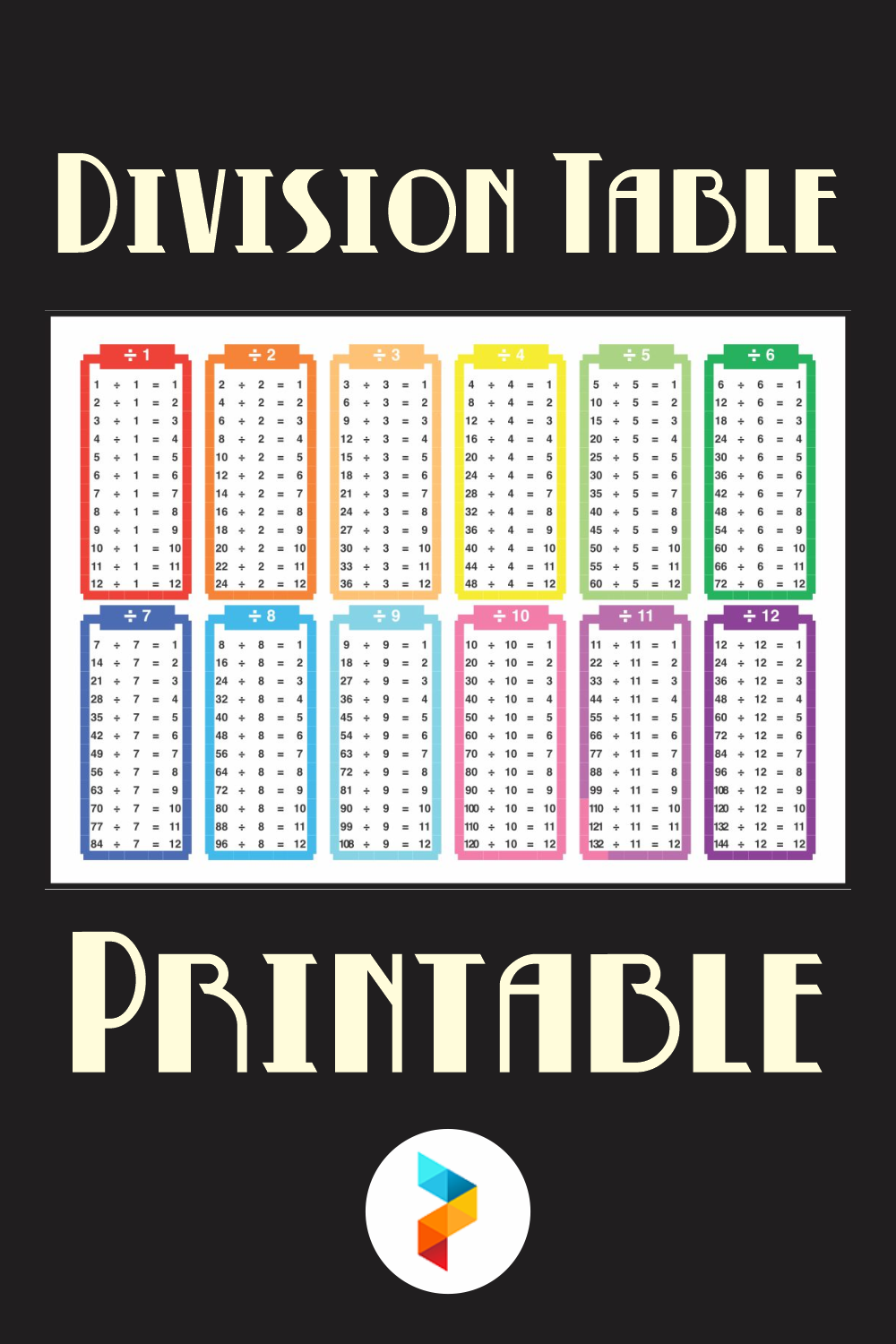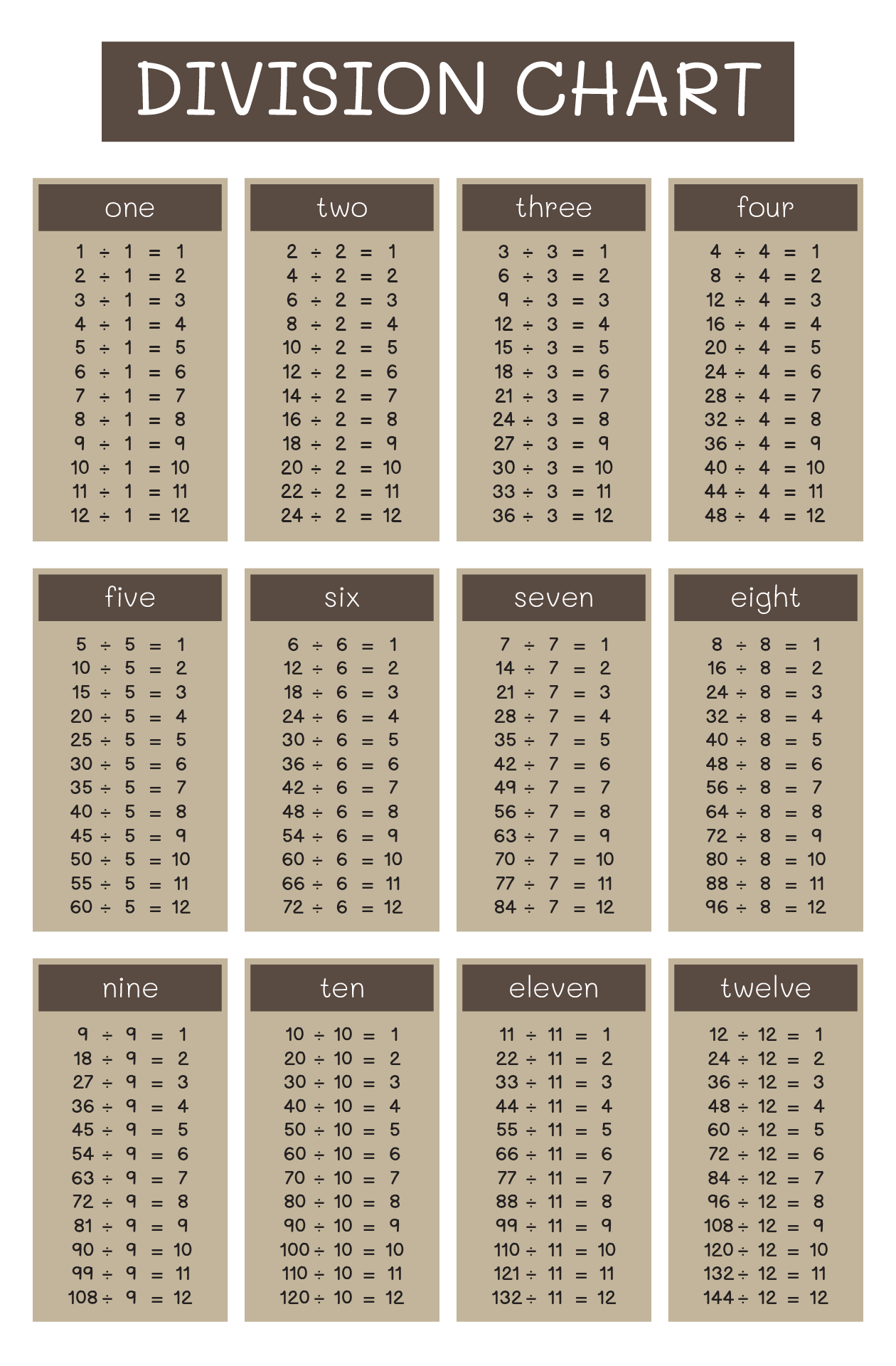### What is a division table?

The division table is a chart that consists of lists of divisions. Usually, this table is used to help kids memorize and understand the process and the result of divisions. It commonly shows the divisions of numbers 0 to 10. However, we can also find lists of 0 to 12 divisions. Actually, the division table is a reversed form of the multiplication table.

### How to complete the division table?

Here’s how to complete a division table:

1.       The division table consists of a factor of 1 to 10 or 1 to 12

2.       Start the division number by picking 0. This number is chosen as the number we want to be divide by

3.       Then, pick the divisor. We can choose 1 as the first divisor

4.       We can find the result equals 0

5.       The first factor of the division table is 1. Make sure numbers that we want to be divided by are multiplications of 1.

6.       Therefore, on multiplications of 1, the numbers that will be divided by start from 0, 1, 2, 3, to 10 or 12.

7.       The next list of the division table are factors of 2

8.       Hence, the numbers that will be divided by are 0, 2, 4, 6, to 20 or 24

9.       We can repeat this to lists of the multiplication’s numbers of 3 to 10 or 12.

10.   Remember, from the lists of tables, the divisors are factors of 1, which is 1, 2, 3….10 or 12.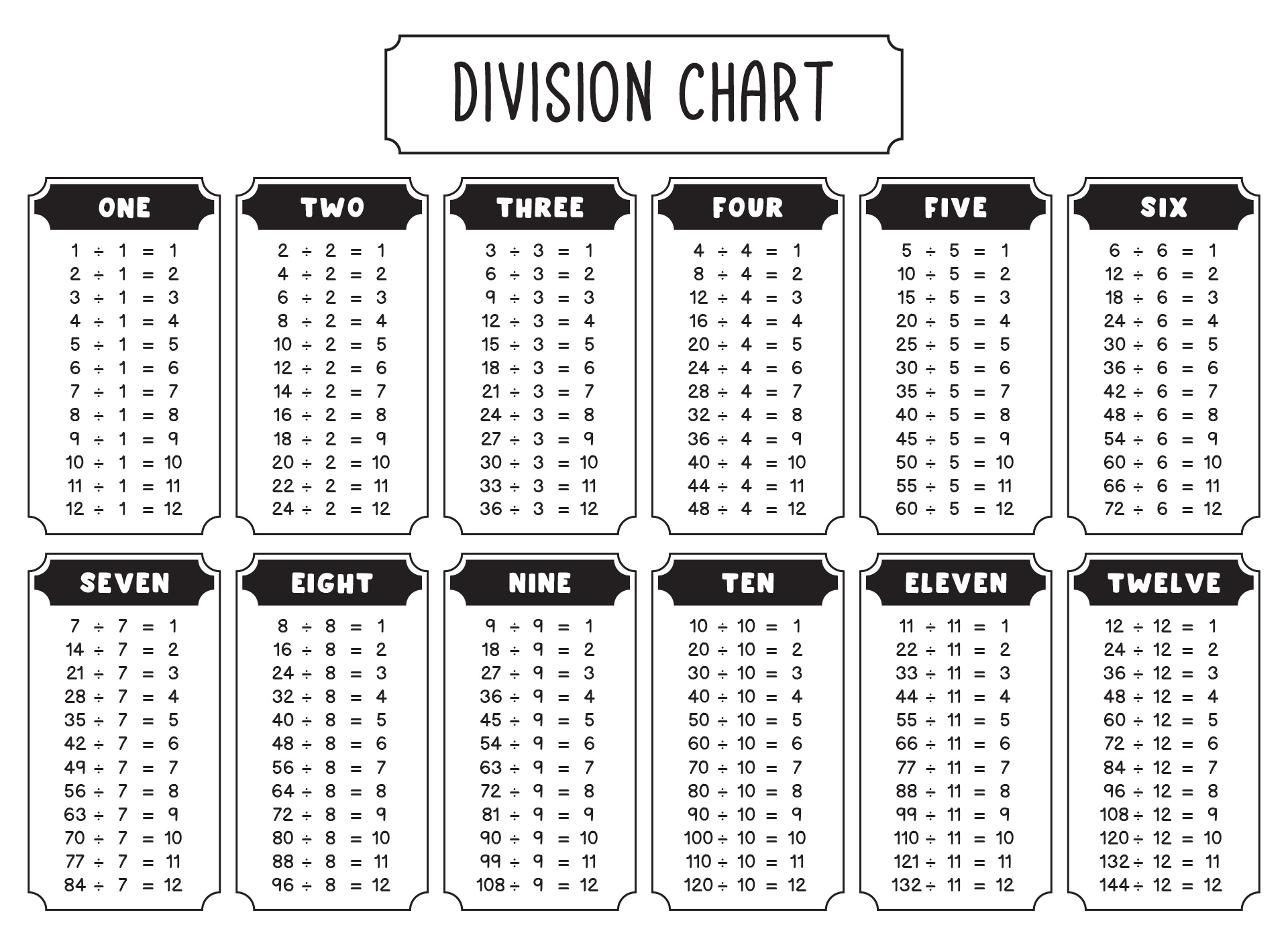We also have more printable other you may like:
144 Miniatures Printables
Printable Cookbook Covers To Print
Printable Medical Excuse Forms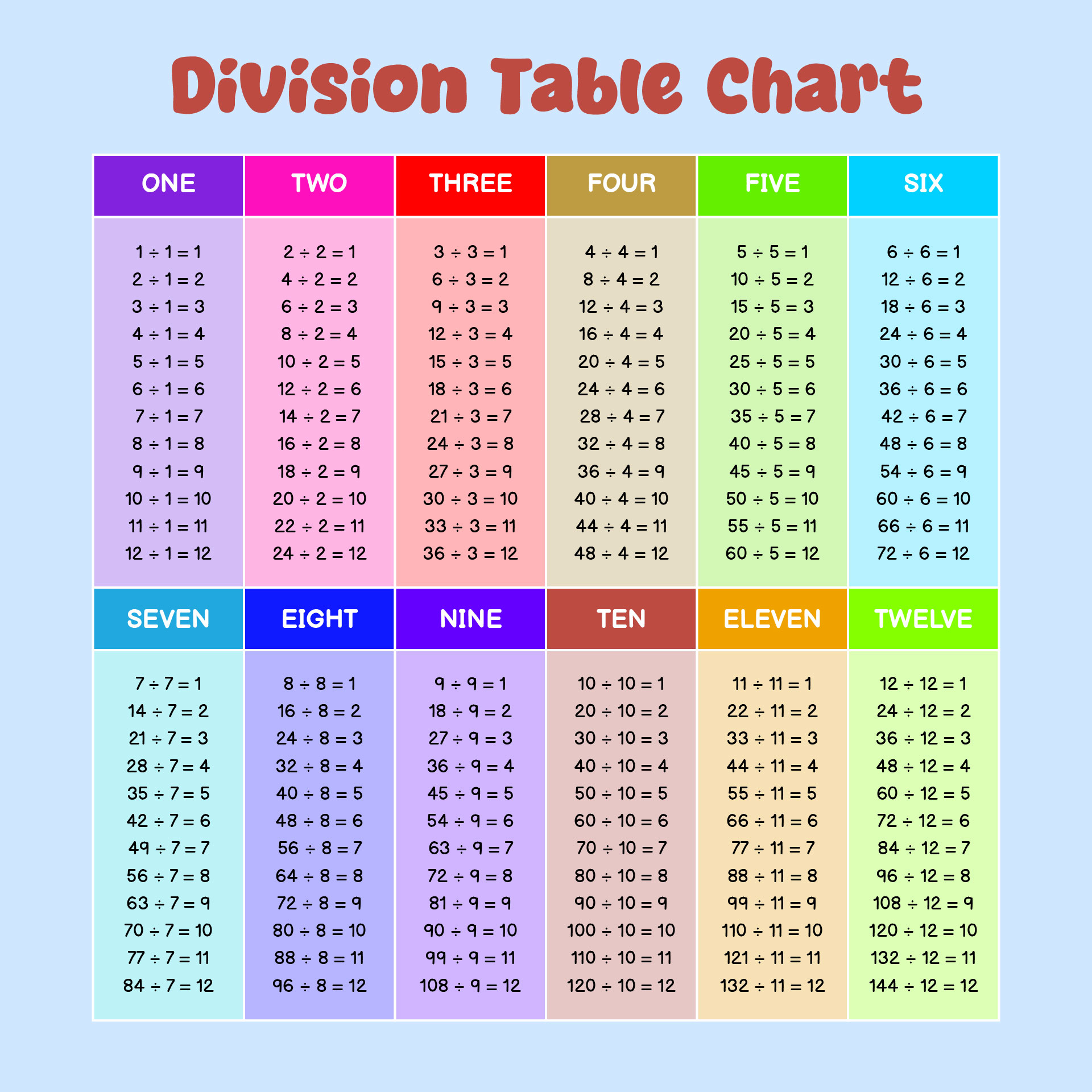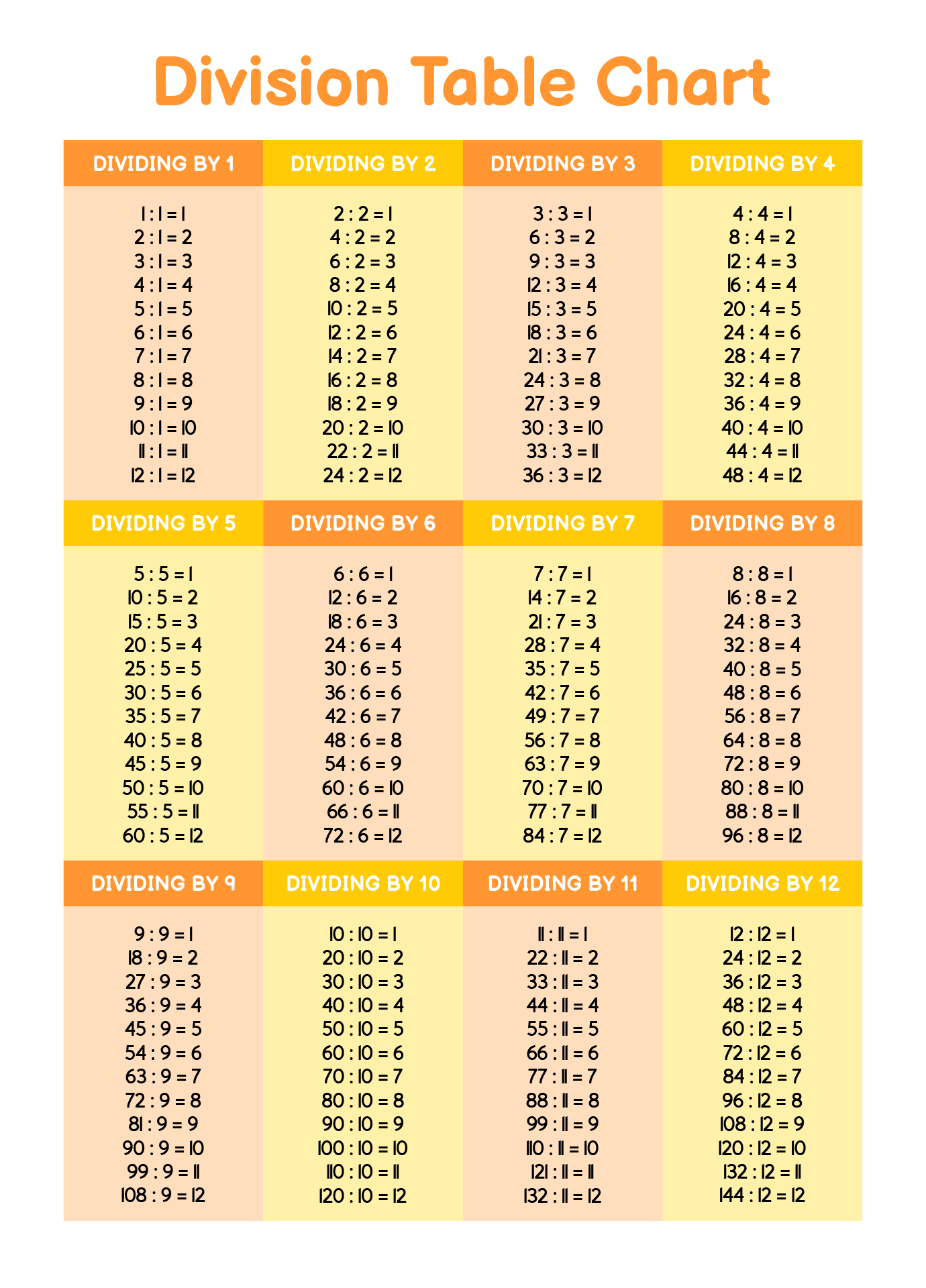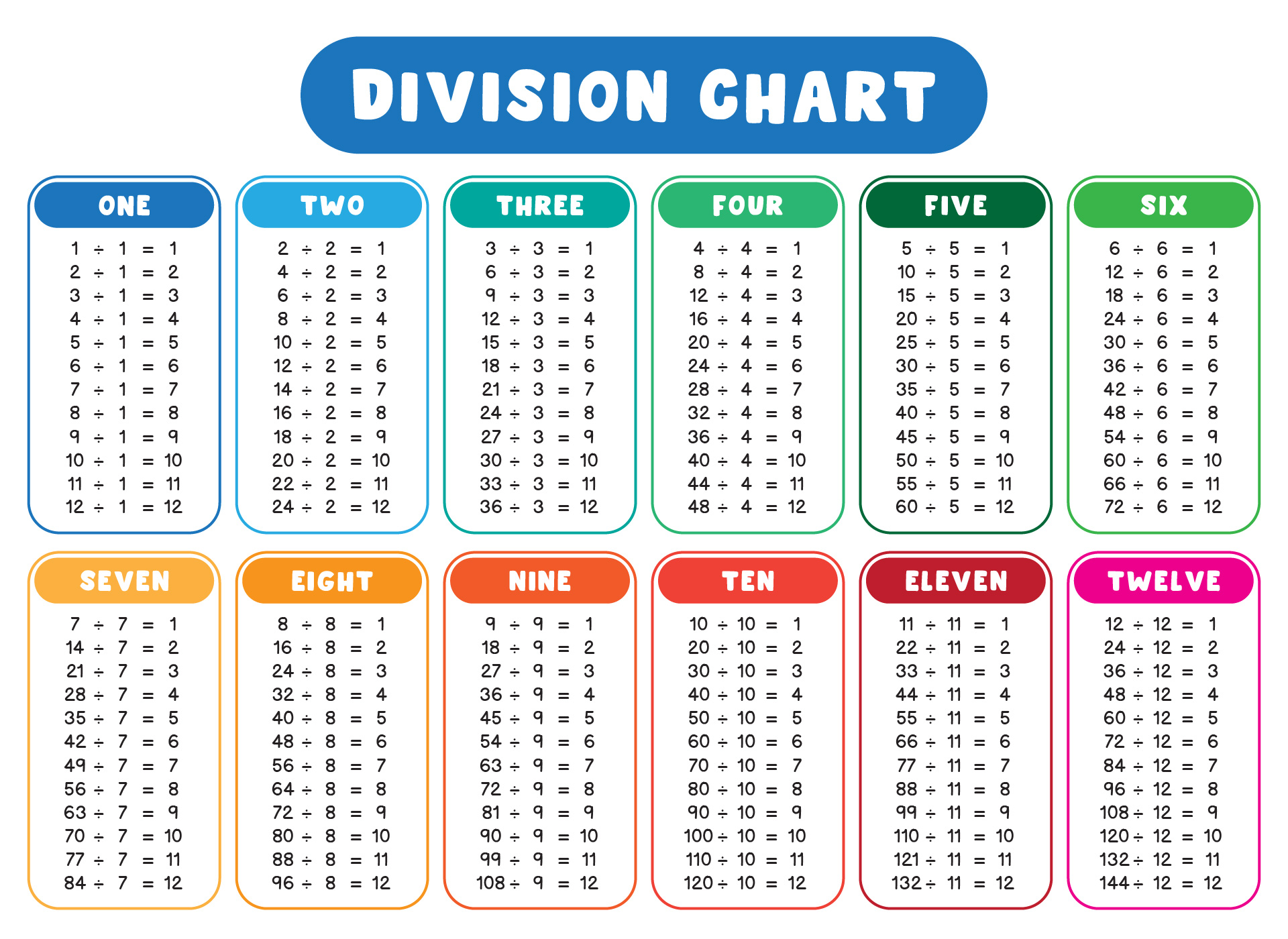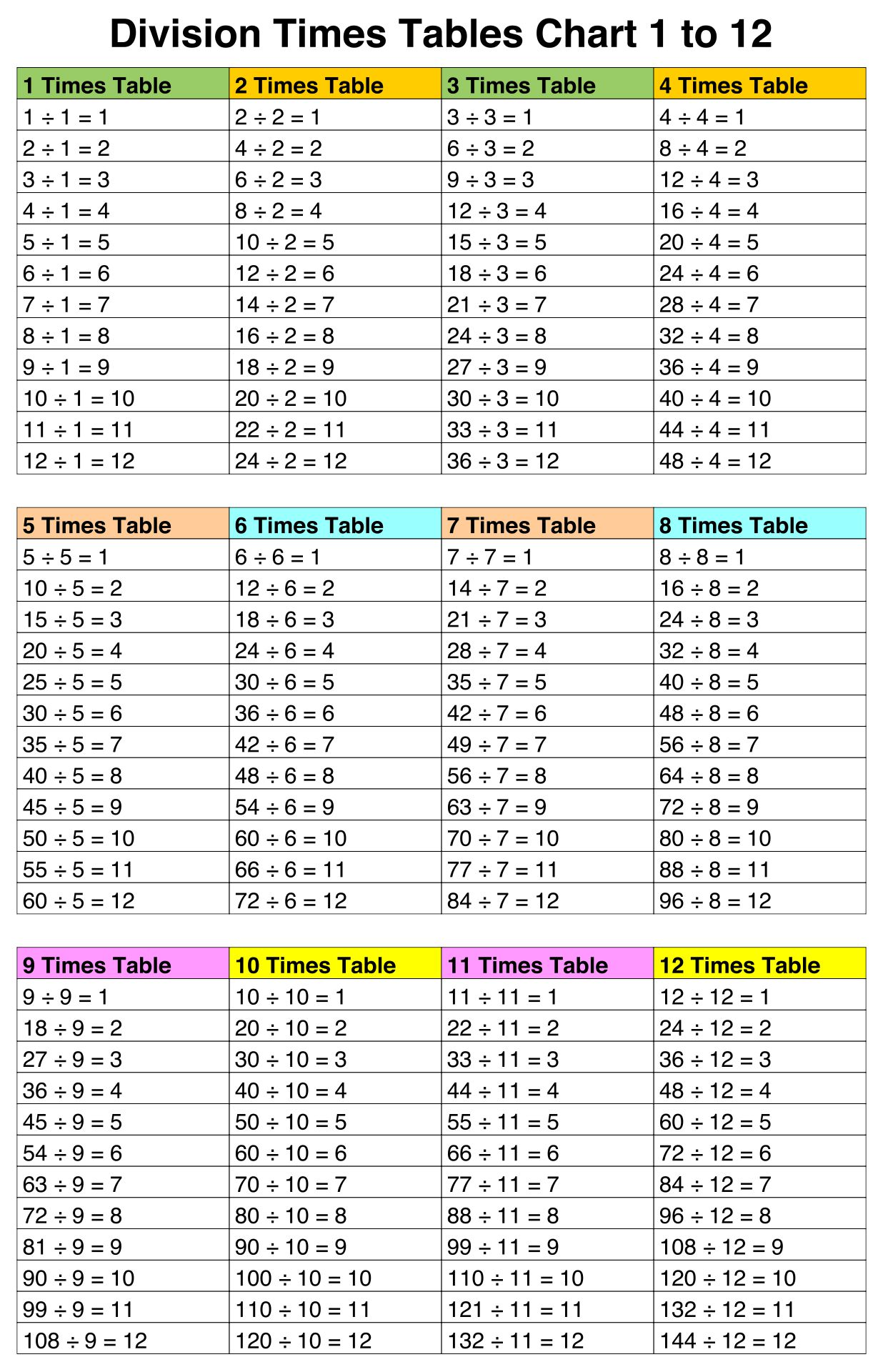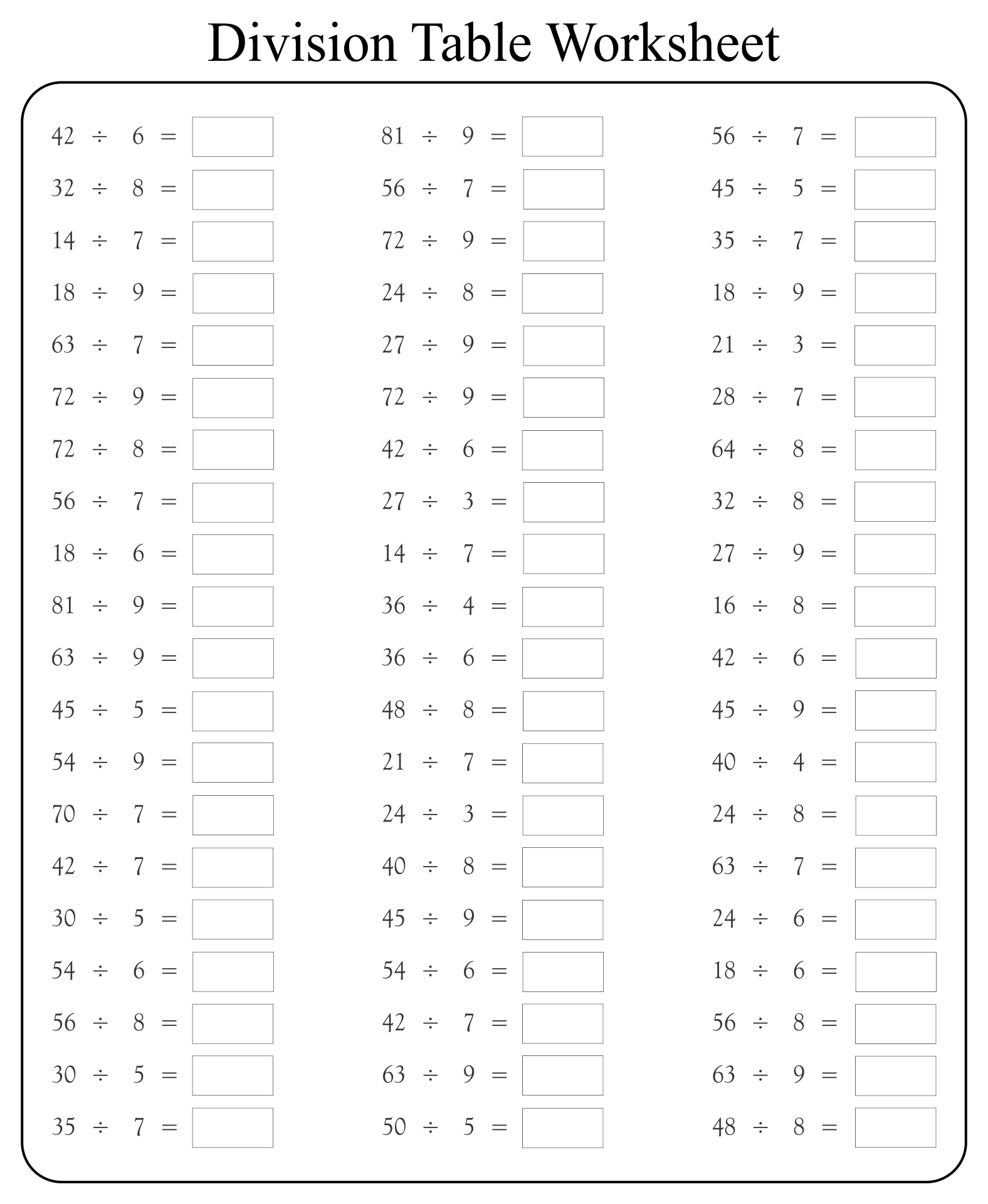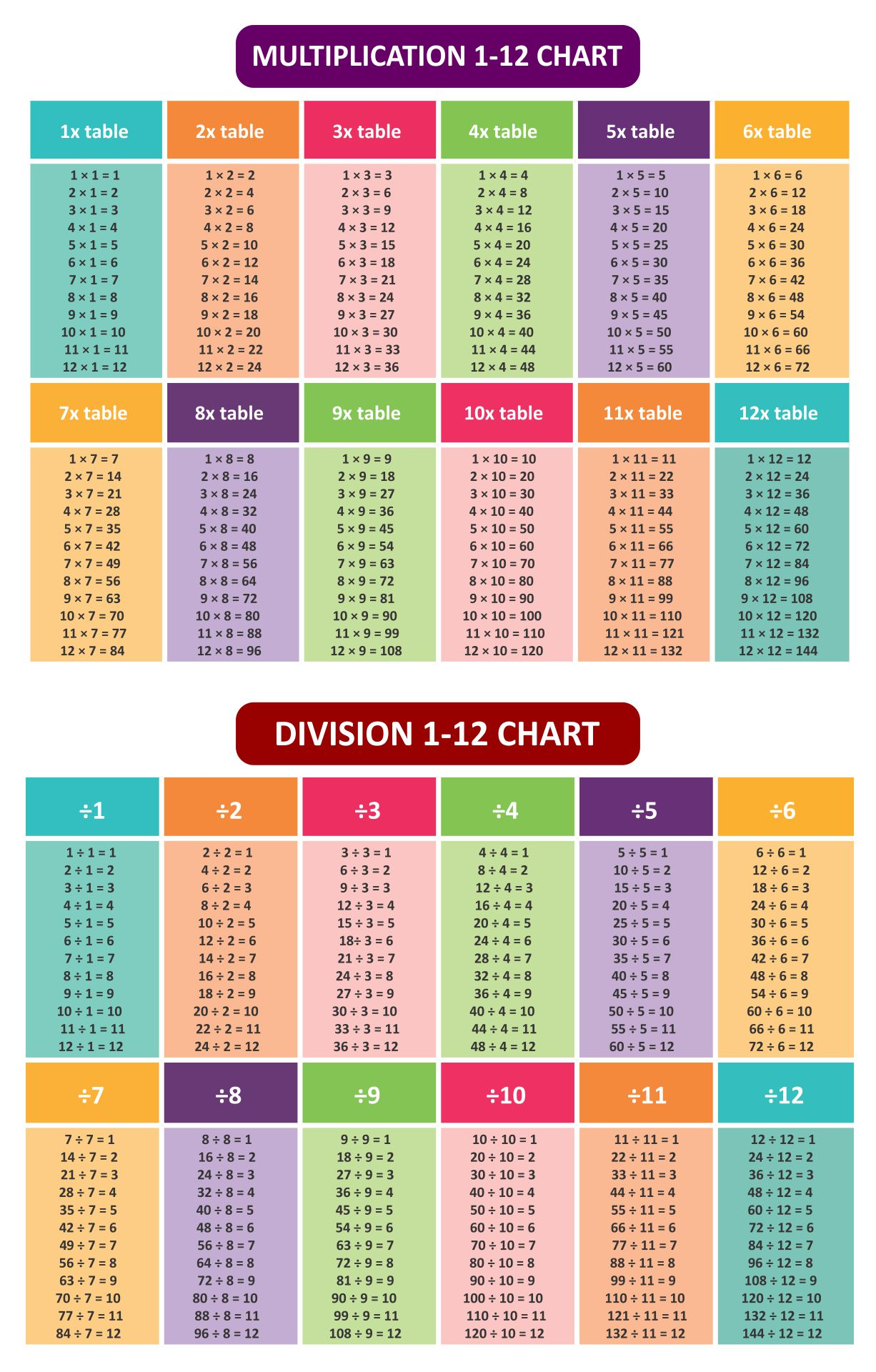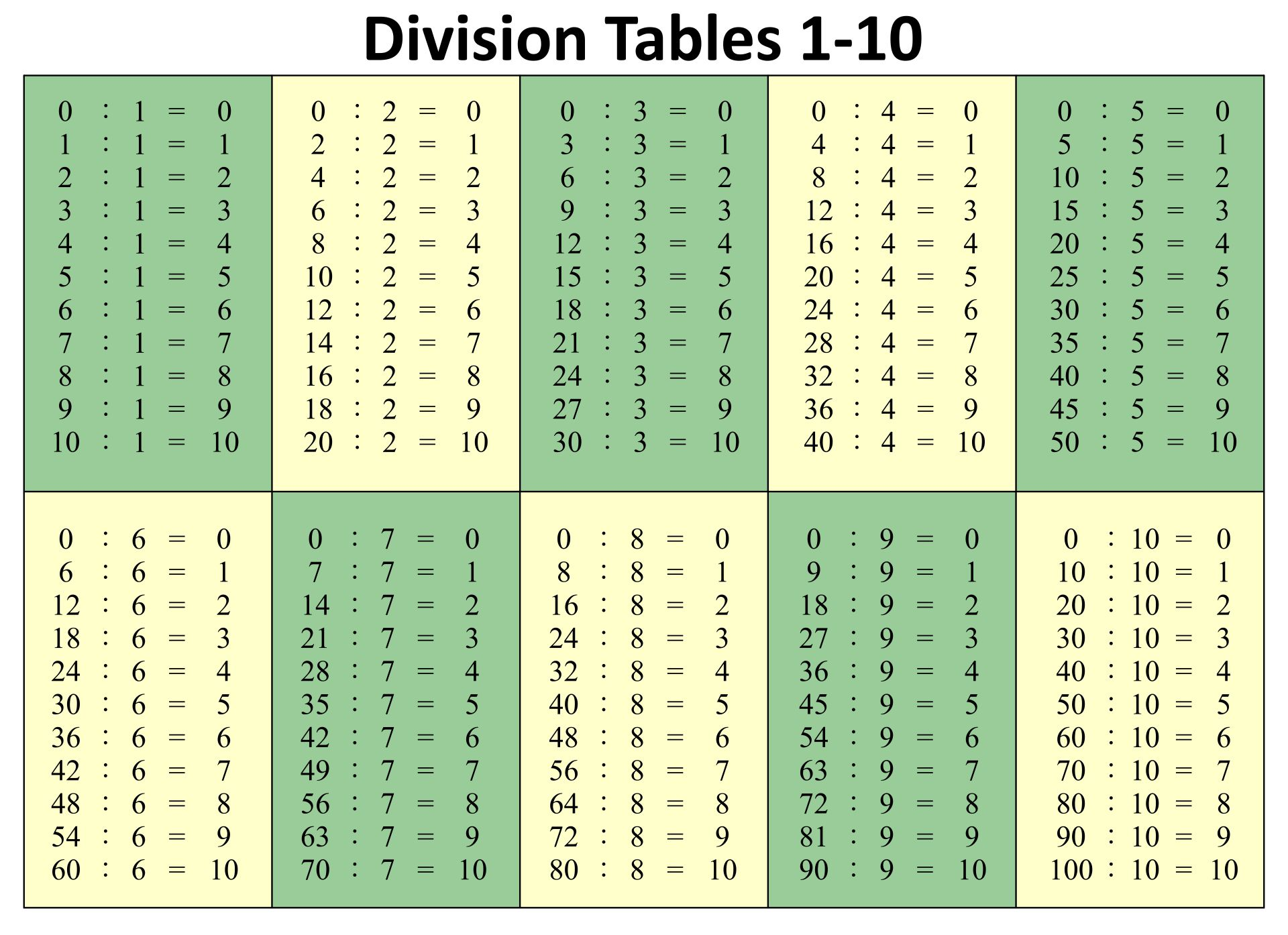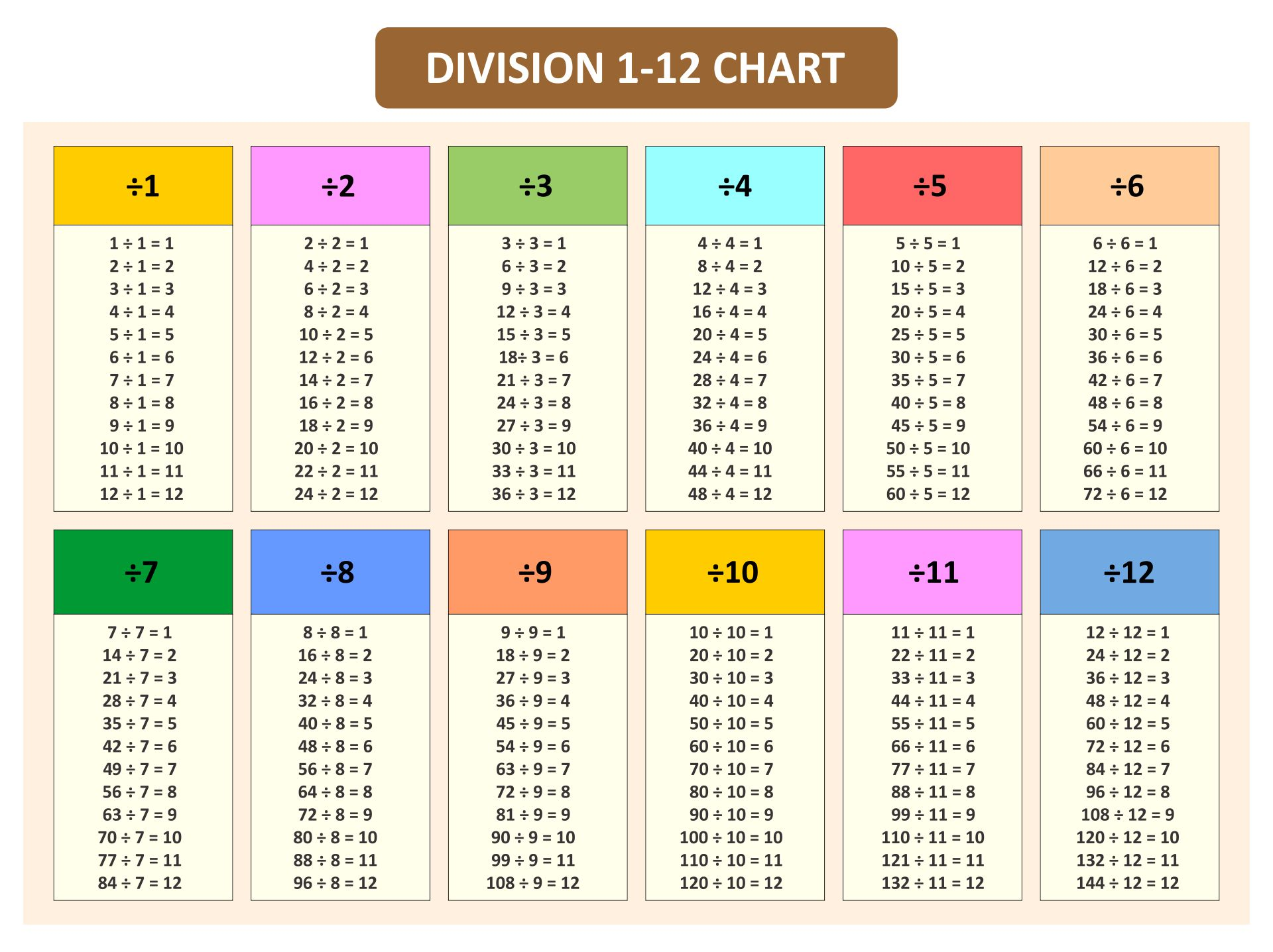### What are the division formulas?

Divisions are actually reversed forms of multiplication. If we want to find the answer of 3×4, we can solve it by adding 3 as many as four times. Thus, we can elaborate it like this: 3+3+3+3. It’s similar with divisions. If we want to find the result of 12÷4, we can do the problem by using subtraction. Remember, to find the correct answer, we have to subtract it until the answer reaches 0. For instance, 12÷4 is similar to 12−4=8, 8−4=4, then 4−4=0. We can conclude that there are subtractions as many as three times. Hence, 12÷4 equals 3.

Generally, there are three main points of a division. They consist of a dividend, a divisor, and a quotient. Dividend is the number that will be divided. Divisor is the number that is used to divide. Lastly, quotient is the number of the result of the divisions. So, we can conclude that:

Dividend÷divisor=quotient.

However, there are certain cases of this operation.

1.       If any number (dividend) is divided by one, then the answer equals the same number.

2.       If any number is divided by zero, then the answer is unidentified. Actually, there is no number that can be divided by zero.

3.       If dividend and divisor are the same number, then the answer is always one.